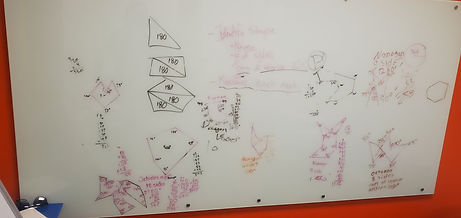## Mr. Jake

### Target 1​

###### Lesson Type:

Continuation

Geometry

:

Shape

Find the sum of the interior angles of convex polygons.

###### 1:

Identify the interior angles of convex polygons.

###### 2:

Explain that polygons have the same number of interior angles as sides.

###### 3:

Discover the Triangle Sum Theorem, which states that the sum of the measures of the interior angles in any triangle will always be 180°.

###### 4:

Use the Triangle Sum Theorem to make conclusions about the interior angles of other polygons.

###### 5:

Use understandings of the sum of interior angles of polygons to solve for an unknown angle measurement or a variable.

7th

###### Vocabulary:

Polygon, Angle, Interior, Side

Activities:

Determined the sum of the interior angles of different polygons

Used the sum of the interior angles of a polygon(s) to answer questions about unknown angles

Determined the missing angle in a polygon given the other angles### Home Exploration

###### Guiding Questions:## Absent Students:

### Target 2

:

###### Vocabulary:

Activities:### Home Exploration

###### Guiding Questions:### Target 3

:

###### Vocabulary:

Activities:### Home Exploration